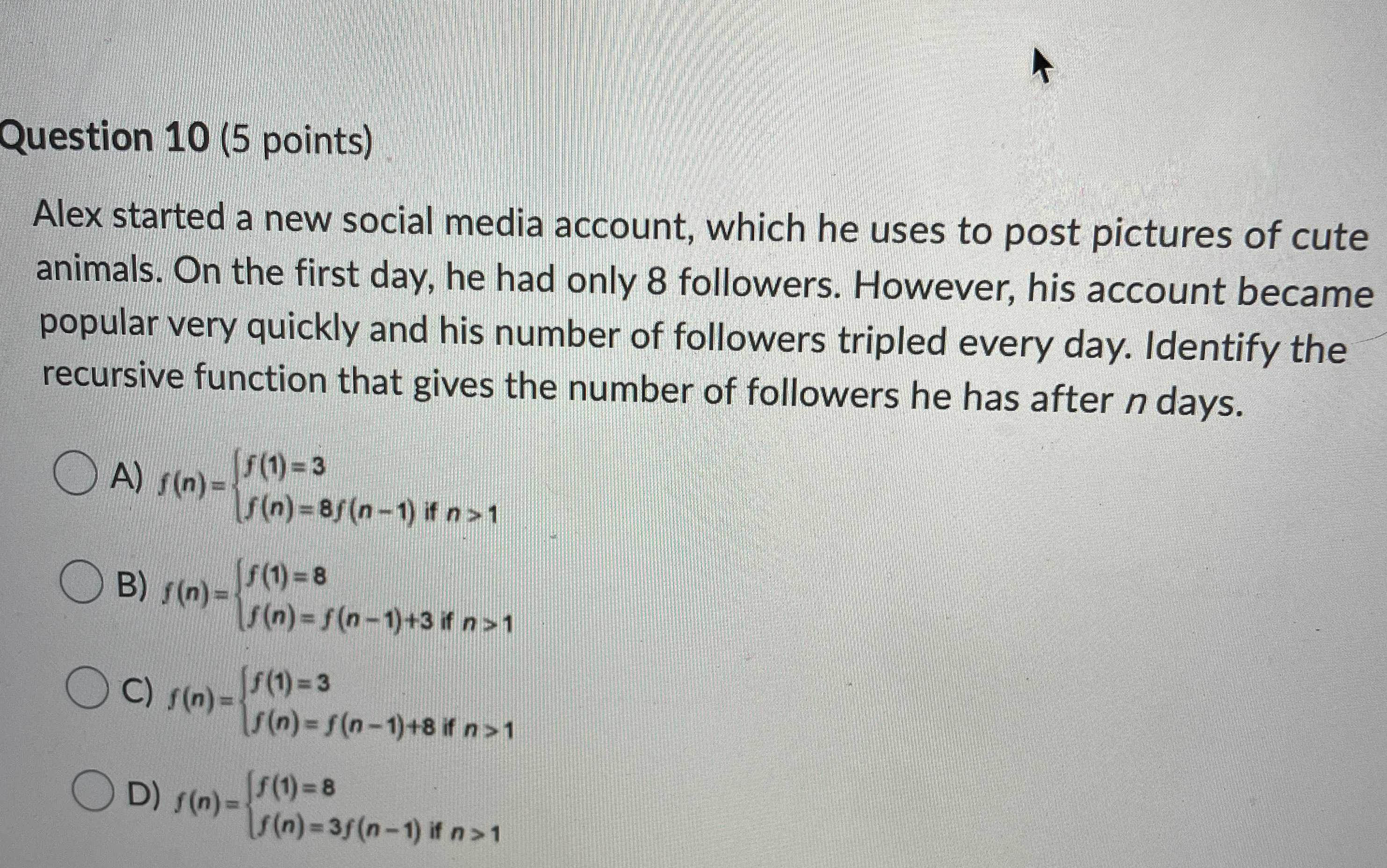### Still have math questions?

Algebra
QuestionAlex started a new social media account, which he uses to post pictures of cute animals. On the first day, he had only $$8$$ followers. However, his account became popular very quickly and his number of followers tripled every day. Identify the recursive function that gives the number of followers he has after $$n$$ days.

A) $$f ( n ) = \left\{ \begin{array} { l } { f ( 1 ) = 3 } \\ { f ( n ) = 8 f ( n - 1 ) if n > 1 } \end{array} \right.$$

B) $$f ( n ) = \left\{ \begin{array} { l } { f ( 1 ) = 8 } \\ { f ( n ) = f ( n - 1 ) + 3 if n > 1 } \end{array} \right.$$

C) $$f ( n ) = \left\{ \begin{array} { l } { f ( 1 ) = 3 } \\ { f ( n ) = f ( n - 1 ) + 8 if n > 1 } \end{array} \right.$$

D) $$f ( n ) = \left\{ \begin{array} { l } { f ( 1 ) = 8 } \\ { f ( n ) = 3 f ( n - 1 ) if n > 1 } \end{array} \right.$$